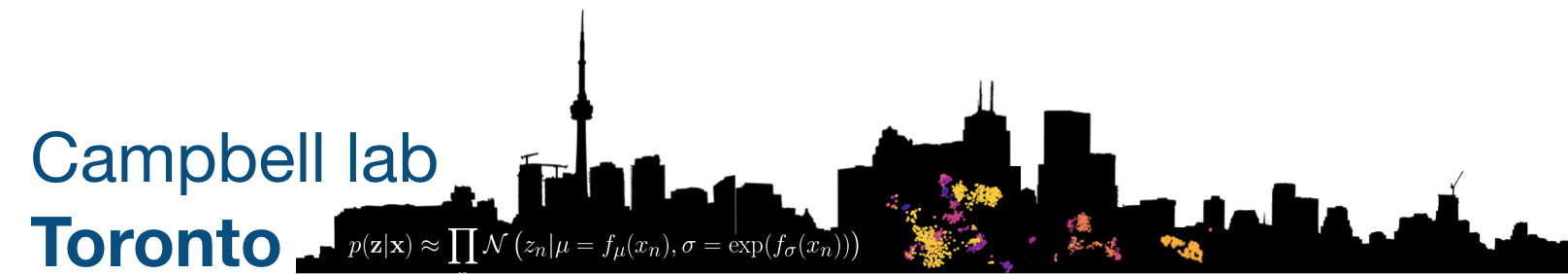#### Foundational Computational Biology I-II (2022)

Graduate course, Department of Molecular Genetics, University of Toronto. Note: only lectures given by me are listed below.

##### Lectures
• [1.1.2] An introduction to reproducible computational research [slides]
• Why work reproducibly?
• Pseudorandom number generation and seeds
• An introduction to Snakemake
• [1.3.2] An introduction to supervised machine learning [slides]
• An overview of supervised learning
• Linear regression models
• Loss optimization via gradient descent
• Classification with logistic regression
• More complex models
• Train/test splits
• Model complexity: overfitting and underfitting
• Penalized regression
• [2.1.1] Unsupervised learning: continuous latent variable models [slides]
• Historical perspective
• Principal component analysis and pPCA
• Non-negative matrix factorization
• Nonlinear methods: tSNE & autoencoders
• [2.1.2] Unsupervised learning: mixture models [slides]
• Clustering from a probabilistic perspective
• Gaussian Mixture Models
• Expectation-maximization
• Model selection
• [2.3.1] Introduction to Bayesian inference [slides]
• Re-introduction to Bayes rule
• Sampling methods: Gibbs sampling, Metropolis Hastings
• Variational inference
• An introduction to probabilistic programming languages & STAN
• [2.3.2] Introduction to deep learning [slides]
• The perceptron, multi-layer perceptrons